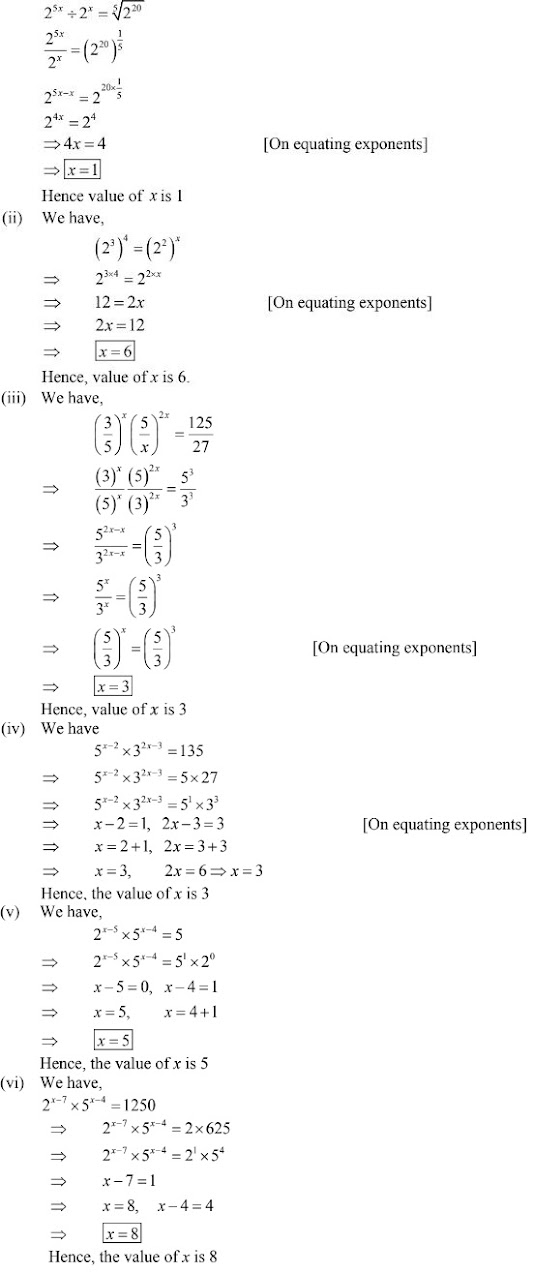# Chapter 2 Exponents of Real Numbers RD Sharma Solutions Exercise 2.1 Class 9 Maths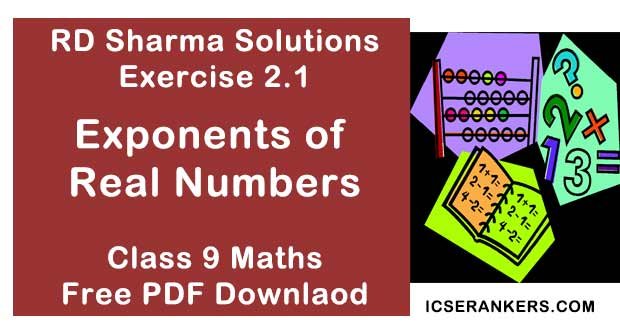Chapter Name RD Sharma Chapter 2 Exponents of Real Numbers Exercise 2.1 Book Name RD Sharma Mathematics for Class 10 Other Exercises No other Exercises Related Study NCERT Solutions for Class 10 Maths

### Exercise 2.1 Solutions

1. Assuming that x, y, z are positive real numbers, simplify each of the following :
(i) (√(x-3)5
(ii) √(x3y-2 )
(iii) [x-2/3 y-1/2 ]2
(iv) (√x)-2/3 (√y4) ÷ √(xy) -1/2
(v)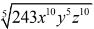(vi) [x-4/y-10]5/4

Solution

(i) We have,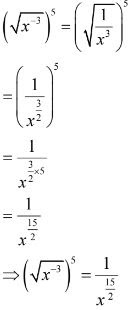(ii) We have,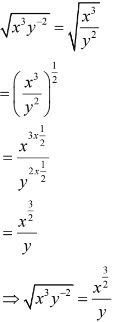(iii) We have,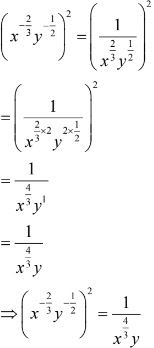(iv) We have,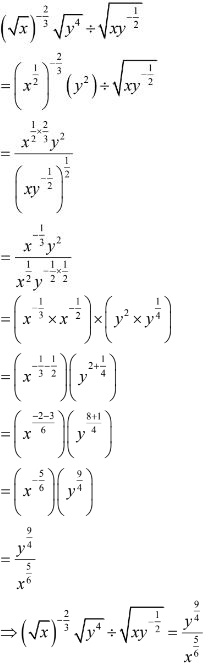(v) We have,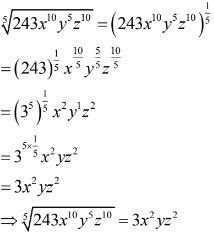(vi) We have,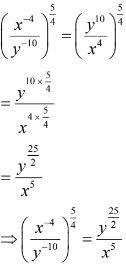2. Simplify :
(i) [16-1/5]5/2
(ii) ∛(342)-2
(iii) (0.001)1/3
(iv) [(25)3/2 × (243)3/5 ]/[(16)5/4 × (8)4/3 ]
(v) (√2/5)8 ÷ (√2/5)13
(vi) [(5-1 × 72)/(52 × 7-4)]7/2 × [(5-2 × 73 )/(53 × 7-5 )]-5/2

Solution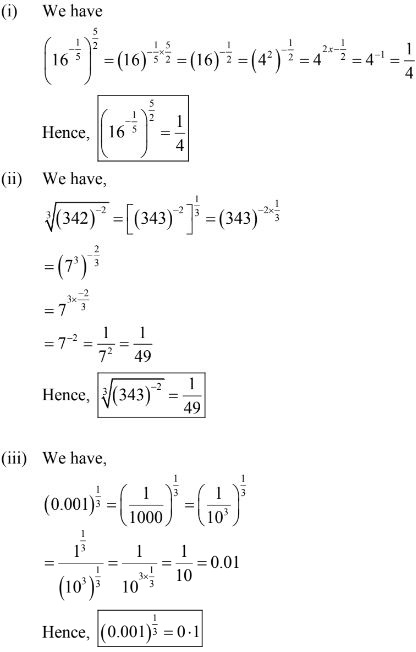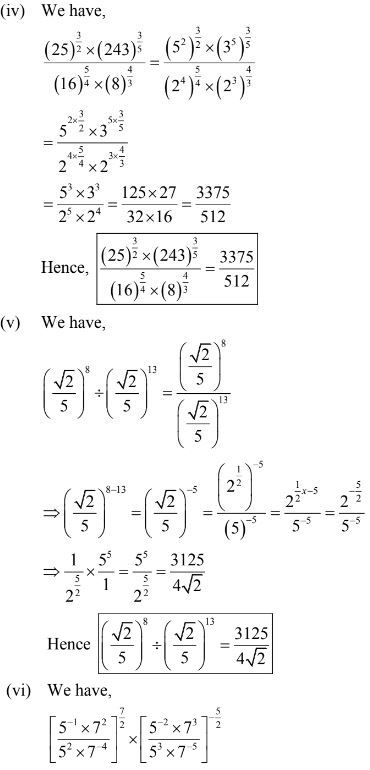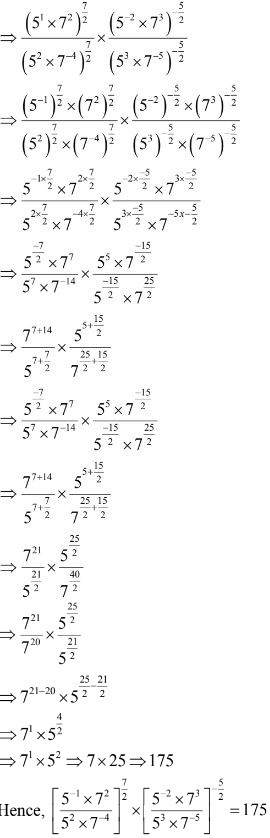3. Prove that :
(i) 93/2 – 3×50 – (1/81)-1/2 = 15
(ii) (1/4)-2 – 3×82/3 × 40 + (9/16)-1/2 = 16/3
(iii) [21/2 × 31/3 × 41/4]/[10-1/5 × 53/5 ] ÷ [34/3 × 5-7/5 ]/[4-3/5 × 6] = 10
(iv) [(0.6)0 – (0.1)-1]/[(3/8)-1 (3/2)3 + (-1/3)-1 ] = -3/2
(v) √(1/4) + (0.01)-1/2 – (27)2/3 = 3/2
(vi) (2n + 2n-1)/(2n+1 – 2n ) = (2n + 2n × 2-1)/(2n × 21 – 2n )
(vii) (64/125)-2/3 + 1/(256/625)1/4 + (√25/∛64)
(viii) (3-3 × 62 × √98)/[52 × ∛1/25 × (15)-4/3 × 31/3 ]
Solution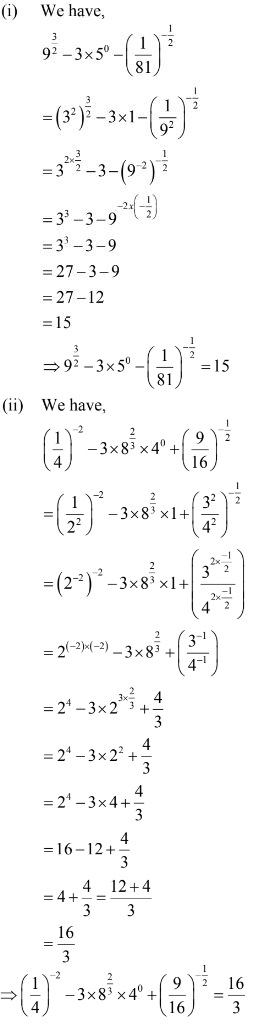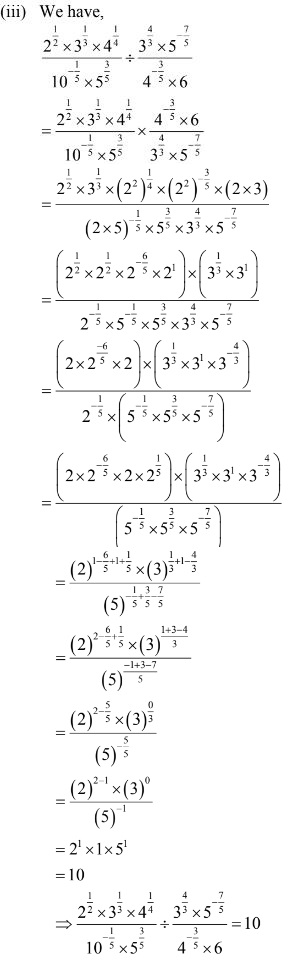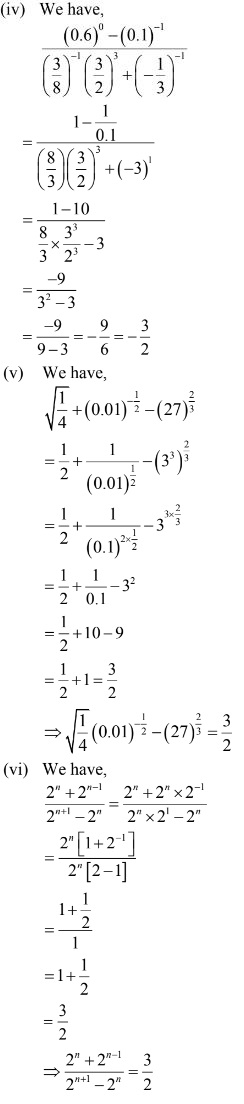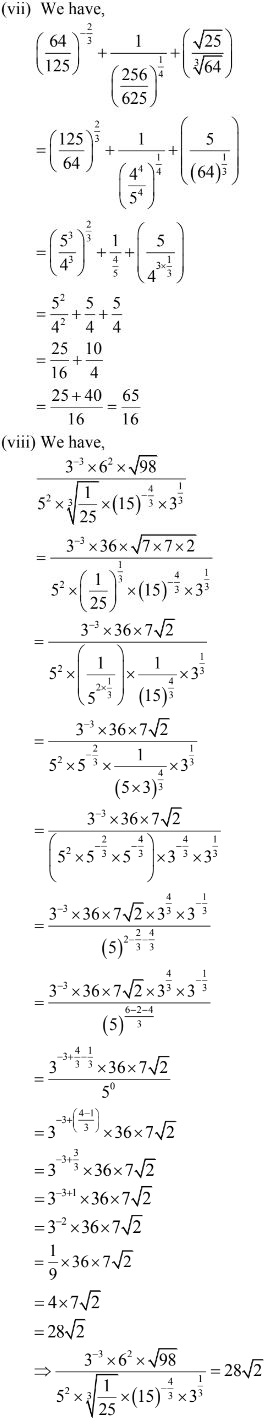4. If 27x  = 9/3x  , find x
Solution
We have,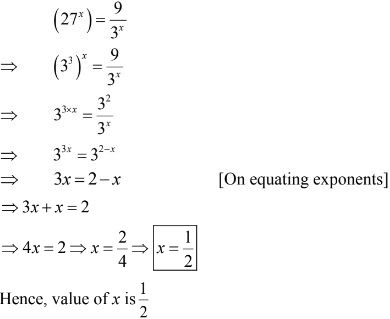5. Find the values of x in each of the following :
(i) 25x ÷ 2x = 5√220
(ii) (23)4 = (22)x
(iii) (3/5)x (5/x)2x = 125/27
(iv) 5x-2 × 32x-3 = 135
(v) 2x-5 × 5x-4 = 5
(vi) 2x-7 × 5x-4 = 1250
Solution
(i) We have